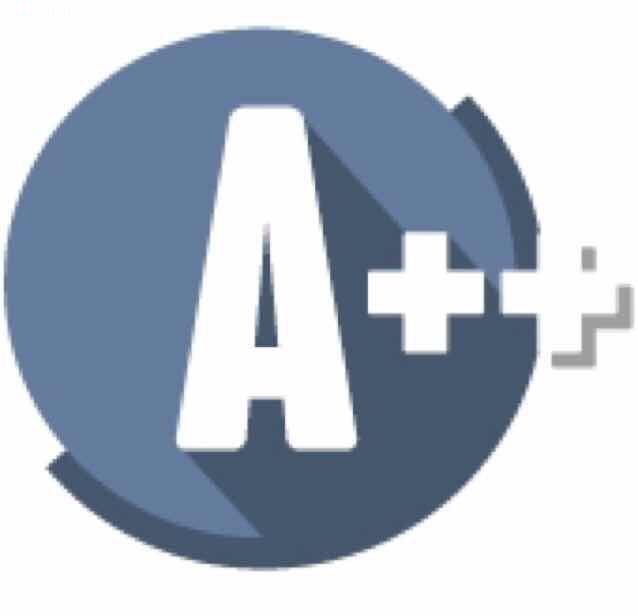## Interviews

#### Check Valid EquationsGiven a series of equations e.g. [A = B, B = D, C = D, F = G, E = H, H = C] and then another series [A != C, D != H, ..., F != A ]

Check whether the equations combined is valid.

For the example given, your program should return 'invalid', because the first series implies that A = C, which contradicts the statement A != C in the second series.

Solution:

This is a 'merge set' question. Given a graph, figure out which nodes belong to the same connected component and put them into a set. Since the input comes in as an edge set, UNION FIND will be a good way to solve this.

Initially every node sources to itself. As we read the statement X = Y, we point the source of Y to the source of X so that they join the same set. After all connected components are sorted out. We check the unequal statements X != Y. If any of the X, Y pairs do share the same source, then X != Y contradicts with the equal statements.

``````public class CheckStatements {
public boolean validStatements(char[][] equal, char[][] unequal) {
int[] sets = new int;
for(int i = 0; i < 26; i++) {
sets[i] = i;
}

for(char[] pair: equal) {
mergeSets(sets, pair - 'A', pair - 'A');
}
for(int i = 0; i < 26; i++) {
findSrc(sets, i);
}

for(char[] pair: unequal) {
if(sets[pair - 'A'] == sets[pair - 'A']) return false;
}
return true;
}

private int findSrc(int[] sets, int i) {
int src = i;
while(src != sets[src]) {
src = sets[src];
}
int tmp;
while (i != sets[i]) {
tmp = sets[i];
sets[i] = src;
i = tmp;
}
return src;
}

private void mergeSets(int[] sets, int a, int b) {
int srcA = findSrc(sets, a);
int srcB = findSrc(sets, b);
sets[srcB] = srcA;
}
}
``````